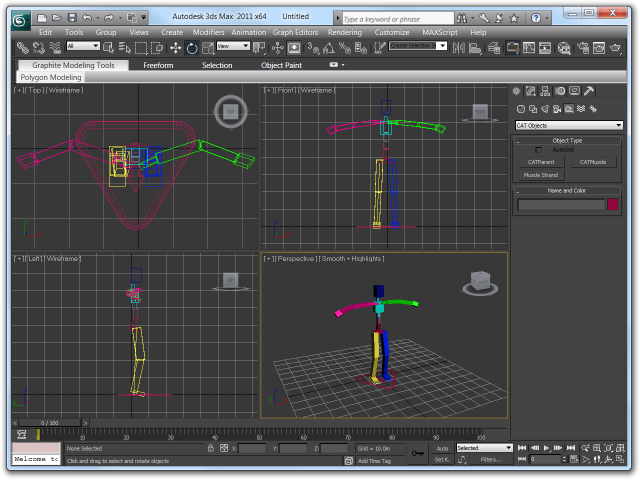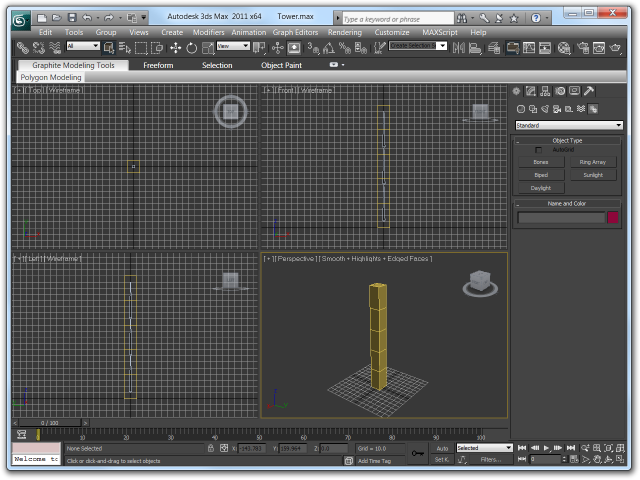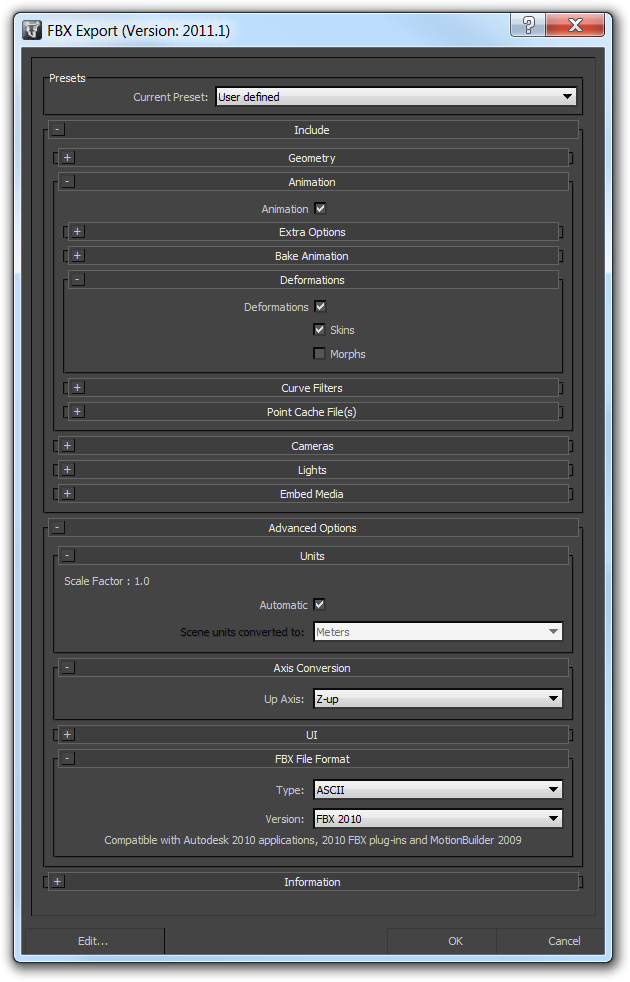# (OLD) Mesh Animation via Bones

This is a follow-up to my previous tutorial How to Rig a Model in 3ds Max that explained the artist’s side of the work. If you haven’t read it yet, I recommend you to start there, if only to know the artist’s side of things.

I originally planned to jump straight into vertex skinning and animation, but after encountering numerous strange issues with XNA’s content processor, affecting my model’s scale and orientation depending on whether meshes had a parent bone assigned and whatnot, I decided to split it into two articles, this first one being about simple multi-mesh animation and publishing a second one once I have vertex skinning fully worked out.

This article explains the process of getting a bone-equipped model animated by programmatically (that means “by code”) moving its bones. This type of animation is ideal for mechanical things like turrets, airplanes or cars where all moving parts are controlled by the player or respond to the environment. It won’t do you much good, however, if you want to animate a walking human or other things which require playback of complicated pre-defined animation cycles.

## The Bind Pose

When you create a model, the pose you initially design it in is called the bind pose. For human models, the bind pose traditionally has been one where the model is standing upright with arms spread:In my previous article, I used a turret model to illustrate some of the finer points (like using the ‘Select Element’ function to get hold of vertices that are difficult to pick because they’re obscured by geometry or other vertices). Since this new article is about code, I’ll be using a non-copyrighted model this time so that you can take a working demo application home with you at the end ;-)

The new model, in its bind pose, is this impressive tower:As you can see, it contains three bones. Each is assigned to the closest 2 vertex groups, so it will behave like a cheaply animated low-poly arm or leg when the bones are moved.

## Exporting to XNA

As I found out, getting the model exported into an XNA game intact can be a bit of a problem. Based on the current moon phase and the contents of your fridge (and perhaps your choice of exporter as well), the model will be scaled by random factors and oriented in random directions.

I ultimately settled on the built-in FBX exporter in 3ds Max because .fbx files have a nice black icon on my system and .x files do not. These are the settings I used:I’m using the FBX 2010 format because there have been a number of drastic changes in the FBX 2011 format. On first look, FBX 2011 seems to be understood by XNA’s content processor without a hitch, though, so don’t let me stop you from using the newer version.

To get the model oriented upright, choose "Z-up" as the Up Axis. I know, in XNA, the Y axis is up, but either the FBX exporter is wrong somewhere or XNA’s content processor is.

The model will also be scaled by a fixed factor of 100 this way, despite the exporter saying "Scale Factor : 1.0". To achieve a perfect 1:1 scaling, you’d have to select "decameters" in the Scene units converted to: combo box, but unless this unit type is added or, better yet, the exporter is fixed, just live with it.

You can either scale the model by a factor of 0.01 in the content processor properties or edit the .fbx file (if you select the Ascii .fbx format) to change the UnitScaleFactor and OriginalUnitScaleFactor to 1.0 like this:

```GlobalSettings:  {
Version: 1000
Properties60:  {
Property: "UpAxis", "int", "",2
Property: "UpAxisSign", "int", "",1
Property: "FrontAxis", "int", "",1
Property: "FrontAxisSign", "int", "",-1
Property: "CoordAxis", "int", "",0
Property: "CoordAxisSign", "int", "",1
Property: "OriginalUpAxis", "int", "",2
Property: "OriginalUpAxisSign", "int", "",1
Property: "UnitScaleFactor", "double", "",1
Property: "OriginalUnitScaleFactor", "double", "",1
Property: "AmbientColor", "ColorRGB", "",0,0,0
Property: "DefaultCamera", "KString", "", "Producer Perspective"
Property: "TimeMode", "enum", "",6
Property: "TimeSpanStart", "KTime", "",0
Property: "TimeSpanStop", "KTime", "",153953860000
}
}
```

## Rendering

A small hurdle you have to take is that XNA’s ContentManager class caches loaded assets – if you call `Load()` with the same path twice, the same asset is returned again from its cache. Thus, if you modify any of the data in your Model, all future instances of that Model will contain those modifications, too.

Most character animation libraries have the concept of a "model instance", in other words, one appearance of the model in a game — the "model" holds the data that remains unchanged between all appearances of the model (textures, vertex buffers, and so on) while the "model instance" stores the data specific to one appearance such as the current pose and animation sequence being played.

It’s possible to do the same in XNA if you take care of the drawing code yourself. Just make sure to not modify any data stored in the `Model` class:

```/// <summary>Maintains an individual appearance of a model in the game</summary>
public class ModelInstance {

/// <summary>Initializes a new model instance</summary>
/// <param name="model">Model of which an instance will be created</param>
public ModelInstance(Model model) {
this.model = model;

int boneCount = model.Bones.Count;

this.boneTransforms = new Matrix[boneCount];
for(int index = 0; index < boneCount; ++index) {
this.boneTransforms[index] = Matrix.Identity;
}

this.world = Matrix.Identity;
}

/// <summary>
///   World matrix controlling the position and orientation of the entire model
/// </summary>
public Matrix World {
get { return this.world; }
set { this.world = value; }
}

/// <summary>Relative transforms of the individual bones in the model</summary>
public Matrix[] BoneTransforms {
get { return this.boneTransforms; }
}

/// <summary>Draws the model in its current pose at its current location</summary>
/// <param name="camera">Camera the model is being viewed from</param>
public void Draw(Camera camera) {
// ? ? ?
}

/// <summary>Model this renderer is drawing</summary>
public Model model;

/// <summary>Position and orientation of the model in the world</summary>
private Matrix world;

/// <summary>Transforms being applied to this model instance only</summary>
private Matrix[] boneTransforms;

}
```

(The `Camera` class I used in above code snippet is just a small class holding the View and Projection matrices - managing the viewer's location, orientation and field of view.)

### Replicating Model.Draw()

I'll build the draw method step by step: as you can see, the `ModelInstance` class keeps its own array with the current orientations of each bone. If you called the Model.Draw() method, it would use Model.CopyAbsoluteBoneTransformsTo() method which relies on the current transform matrices stored in the bones themselves, so I work around this by performing the work done by this method myself.

First, to achieve the equivalent of `Model.Draw()`, add this to the `ModelInstance` class:

```/// <summary>Initializes a new model instance</summary>
/// <param name="model">Model of which an instance will be created</param>
public ModelInstance(Model model) {
// ... existing code ...
this.absoluteBoneTransforms = new Matrix[boneCount];
}

// ... existing methods ...

/// <summary>Draws the model in its current pose at its current location</summary>
/// <param name="camera">Camera the model is being viewed from</param>
public void Draw(Camera camera) {

// Calculate the absolute (model space) transformations of each bone
calculateAbsoluteBoneTransforms();

int meshCount = this.model.Meshes.Count;
for (int meshIndex = 0; meshIndex < meshCount; meshIndex++) {
ModelMesh mesh = this.model.Meshes[meshIndex];
int parentBoneIndex = mesh.ParentBone.Index;

int effectCount = mesh.Effects.Count;
for (int effectIndex = 0; effectIndex < effectCount; effectIndex++) {
Effect effect = mesh.Effects[effectIndex];
if (effect == null) {
continue; // Model.Draw() would throw an exception in this case
}

// Hand the mesh's transformation matrices to the effect
IEffectMatrices matrices = effect as IEffectMatrices;
if (matrices != null) {
matrices.World = this.absoluteBoneTransforms[parentBoneIndex] * world;
matrices.View = camera.View;
matrices.Projection = camera.Projection;
}
}

mesh.Draw();
}
}

/// <summary>Calculates the absolute bone transformation matrices in model space</summary>
private void calculateAbsoluteBoneTransforms() {

// Obtain the local transform for the bind pose of all bones
this.model.CopyBoneTransformsTo(this.absoluteBoneTransforms);

// Convert the relative bone transforms into absolute transforms
ModelBoneCollection bones = this.model.Bones;
for (int index = 0; index < bones.Count; ++index) {
this.absoluteBoneTransforms[index] = bones[index].Transform;

// Content processors sort bones so that parent bones always appear
// before their children, thus this works like a matrix stack,
// resolving the full bone hierarchy in minimal steps.
ModelBone bone = bones[index];
if (bone.Parent != null) {
int parentIndex = bone.Parent.Index;
this.absoluteBoneTransforms[index] *= this.absoluteBoneTransforms[parentIndex];
}
}

}

// ... existing fields ...

/// <summary>Absolute transform matrices for all bones in model space</summary>
/// <remarks>
///   The contents of this field are recreated on-the-fly during each Draw() call,
///   but to avoid feeding the gargabe collector by creating a new temporary
///   array each time, we keep reusing this one.
/// </remarks>
private Matrix[] absoluteBoneTransforms;
```

The `calculateAbsoluteBoneTransforms()` method does exactly what the `Model`'s `CopyAbsoluteBoneTransformsTo()` method would do, but stores the resulting absolute model space transforms in a local array instead.

The `ModelInstance.Draw()` method then draws the model's meshes, again, exactly like `Model.Draw()` would do it, but using the stored transformation matrices from the `ModelInstance` class.

### Mesh-based Bone Animation

The bone transformations can now be modified outside of the model class, what remains is to apply them to the model when it is rendered:

```/// <summary>Calculates the absolute bone transformation matrices in model space</summary>
private void calculateAbsoluteBoneTransforms() {

// Obtain the local transform for the bind pose of all bones
this.model.CopyBoneTransformsTo(this.absoluteBoneTransforms);

// Convert the relative bone transforms into absolute transforms
ModelBoneCollection bones = this.model.Bones;
for (int index = 0; index < bones.Count; ++index) {

// Take over the bone transform and apply its user-specified transformation
this.absoluteBoneTransforms[index] =
this.boneTransforms[index] * bones[index].Transform;

// Calculate the absolute transform of the bone in model space.
// Content processors sort bones so that parent bones always appear
// before their children, thus this works like a matrix stack,
// resolving the full bone hierarchy in minimal steps.
ModelBone bone = bones[index];
if (bone.Parent != null) {
int parentIndex = bone.Parent.Index;
this.absoluteBoneTransforms[index] *= this.absoluteBoneTransforms[parentIndex];
}
}

}
```

Unlike in normal algebra, the order in which you perform matrix multiplications is important:

• Multiplying the user-defined bone transformation by the bone's bind pose transformation means that you first create a new rotation matrix on the global rotation axis and then, with the multiplication, transform it by the bind pose orientation, turning it into a rotation that takes place in the bone's local coordinate frame (an X axis rotation on your model's wrist bone would wink the hand up and down relative to the arm's position.)
• Multiplying the bone's bind pose transformation by the user-defined bone transformations means that you take the bone's bind pose orientation and then rotate that on the global rotation axes (so an X axis rotation would wink the wrist towards sky and ground, no matter how the arm is oriented.)
• There's even a third option: if you just switch the operands in the matrix multiplication above, the user-defined transformation is applied on the global axis, but due to the way the `for` loop transforms bones by their parents afterwards, it will still be rotated by the parent bone's transform, effectively rotating the bone on the coordinate frame of its parent frame.

I think only the first variant is useful as it allows the artist to choose the orientation of the bone's coordinate frame - to achieve rotations in the global coordinate frame, for example, the artist would only need to orient the bone upright.